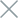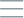• Home
• Tags
• Series
•# Graph Search Algorithm in Java

The Graph Search algorithm is an essential technique in Java programming used to search for vertices or edges within a graph. A graph is a collection of vertices connected by edges. This algorithm is often applied to problems such as finding the shortest path, searching for connections between elements, and analyzing networks.

## How Graph Search Algorithm Works

The Graph Search algorithm has various methods, such as Breadth-First Search (BFS) and Depth-First Search (DFS). Both of these methods involve traversing vertices and edges within the graph to find the target or required condition.

• Breadth-First Search (BFS) traverses the root vertex first and then explores neighboring vertices before moving on to farther vertices.
• Depth-First Search (DFS) explores each vertex and performs a depth-first search until the destination is found or further exploration is not possible.

• Finding connections: This algorithm helps identify connections between vertices in a graph, which is useful for finding shortest paths or relationships between elements.
• Fast search capability: Depending on the graph's structure, the algorithm can quickly search for the target.

• Prone to getting lost: In cases of large and complex graphs, the algorithm may become lost or disoriented, leading to time-consuming searches.

## Example and Explanation

Illustrate the Graph Search algorithm using a Java example that employs the Breadth-First Search (BFS) method to find the shortest path between vertices in a graph.

``````import java.util.*;

public class GraphSearchExample {
// Class implementation of the graph and BFS here...
}

public static void main(String[] args) {
Graph g = new Graph(4);